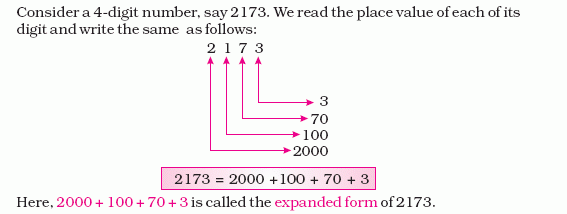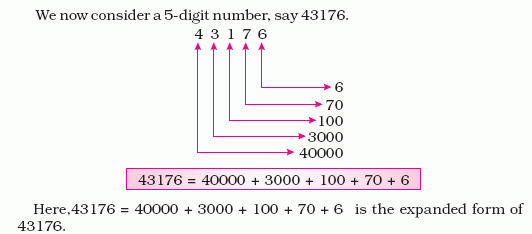kwizNET Subscribers, please login to turn off the Ads!
 Email us to get an instant 20% discount on highly effective K-12 Math & English kwizNET Programs!

#### Online Quiz (WorksheetABCD)

Questions Per Quiz = 2 4 6 8 10

### Grade 4 - Mathematics1.8 Standard/Expanded NotationDirections: Answer the following questions. Also write at least ten examples of your own.
 Q 1: 999=900 + 90 + 9990 + 9900 + 99 Q 2: 457,000=400,000 + 50,000 + 700400,000 + 50,000 + 7400,000 + 50,000 + 7,000 Q 3: 3,079=3000 + 793000 + 70 + 930 + 79 Q 4: 6,987=6,000 + 900 + 876,000 + 900 + 80 + 76,900 + 80 + 7 Q 5: 16,508=10,000 + 6,000 + 500 + 816,000 + 5 + 816,000 + 508 Q 6: 60,040=60,000 + 406,000 + 40600 + 40 Q 7: 23,456=23,000 + 400 + 50 + 623 + 45620,000 + 3,000 + 400 + 50 + 6 Q 8: 700,060=70,000 + 67,000 + 60700,000 + 60 Question 9: This question is available to subscribers only! Question 10: This question is available to subscribers only!

#### Subscription to kwizNET Learning System offers the following benefits:

• Unrestricted access to grade appropriate lessons, quizzes, & printable worksheets
• Instant scoring of online quizzes
• Progress tracking and award certificates to keep your student motivated
• Unlimited practice with auto-generated 'WIZ MATH' quizzes
• Child-friendly website with no advertisements
• Choice of Math, English, Science, & Social Studies Curriculums
• Excellent value for K-12 and ACT, SAT, & TOEFL Test Preparation
• Get discount offers by sending an email to discounts@kwiznet.com

 Quiz Timer